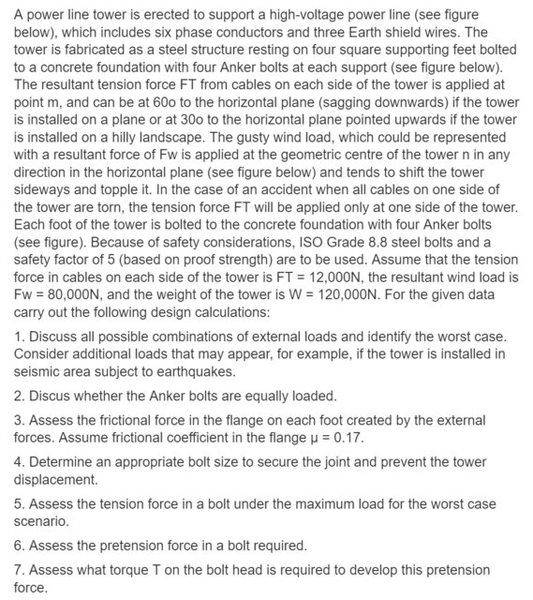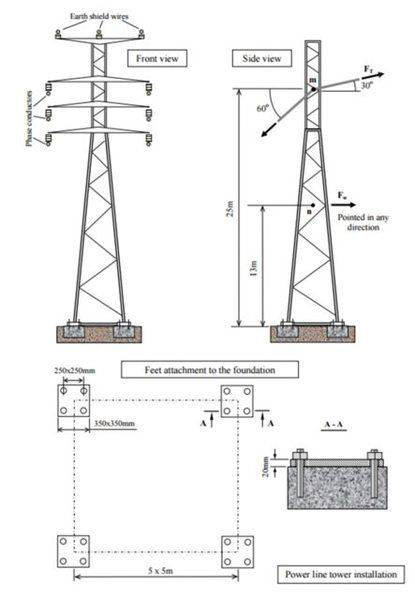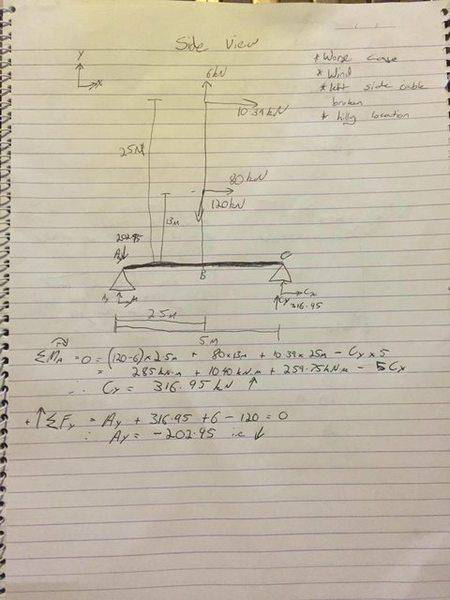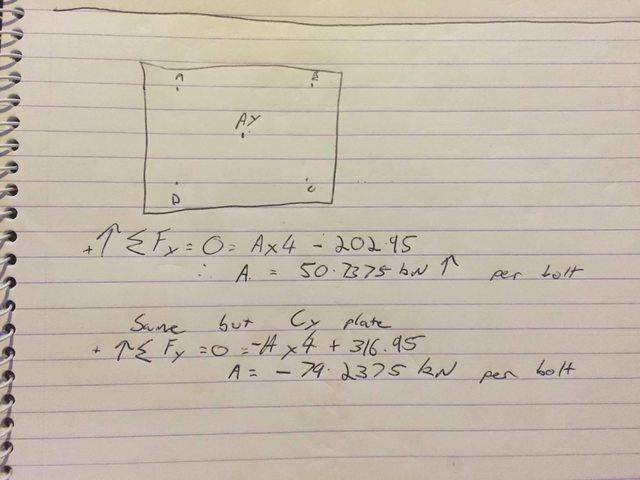# Basic forces and bolts

## Homework Statement## Homework Equations

sum of forces in x and Y = 0
sum of the moments at a point = 0
Ffr = normal force * friction coefficient

## The Attempt at a Solution

Im just trying to make a start, Im struggling to wrap my head around the basics with this question. Do you guys think I have simplified my fbd too much and Im not sure how the force is distrubuted amoung the bolts and the plates and whether to include the distance between bolts in the first fbd or to add it into the calcs of each individual plate.
Not after answers just want to check im on the right pathPhanthomJay
Homework Helper
Gold Member
You have the correct approach, except that there are 2 foundations on the bearing side and 2 on the uplift side, so your numbers for the load on each bolt will need to be divided by 2. But the problem statement is unclear when it mentions all cables torn on one side, so you'd have to multiply the FT load by 6 to account for 6 phase conductors , I guess, and it would be slightly conservative to apply that load all at point m. Looks like earth wires are assumed not to carry any of the unbalanced load.

thanks for the reply, the question is mostly aimed at bolts and threaded fasteners So Im not too worried about the forces on the cables etc I just need to get it right for my calcs. I think what they implied by saying one side broken is either front or back that way the force would be in one direction instead of opposite.

PhanthomJay
Homework Helper
Gold Member
Well ok but you can't find the load on the bolts if the problem is unclear as to what loading to use for the cable tensions. If the resultant load of the cable tensions on one side is 12 kN directed as shown and applied at the center of the tower body at 25 m from the base, then your calculations for the tension in the bolts on the uplift side is off by a factor of 2 because there are 4 foundations not 2.

yer ive fixed that one up thanks

Tom.G
Gold Member
In the real world, at least consider the torque on the tower if cables on one end of the crossarms fail and the diagonal cables on the other end of the crossarms also fail.

What about the frictional force and shear force of the bolts? two methods of thinking either the frictional resistance of the tower is 114*0.17 = 19.38 therefore to reach equilibrium the frictional resistance the combined plates create needs to equal 90.39-19.38 = 71.01kN and each bolt is 71.01/4 = 17.52

Or

friction on left side = (202-57) *0.17 = 24.65 and right side is (316.95 + 57) *0.17 = 63.57 and divide each number by 2 to find for each plate

PhanthomJay